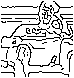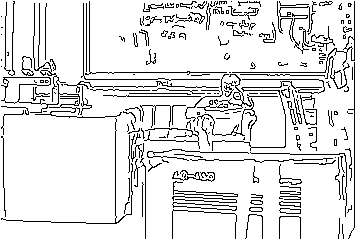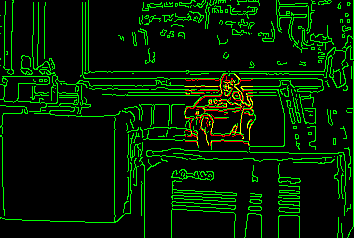### Hausdorff-Based Image Comparison

One of our central areas of research is on developing efficient techniques for comparing binary feature maps (such as intensity edges). The most successful methods that we have develoepd are based on using the generalized Hausdorff measure to compare portions of one image with another. Often the two images are allowed to undergo some kind of geometric transformation in the matching process, and then we are concerned with finding transformations that produce good matchines according to the Hausdorff measure. This measure determines the resemblance of one point set to another, by examining the fraction of points in one set that are near points in the other (and perhaps vice versa). Thus there are two parameters in deciding whether or not two point sets resemble one another - what distance must points be to be considered close together, and what fraction of the points are (at most) this close distance away from points of the other set. This distance measure differs from correspondence-based techniques such as point matching methods and binary correlation, in that there is no pairing of points in the two sets being compared.

For more information on the generalized Hausdorff measure, there is a brief introduction, as well as our paper A Multi-Resolution Technique for Comparing Images Using the Hausdorff Distance. A C implementation of Hausdorff matching (for matching with translation or with translation and scaling) is available as a tar file via ftp.

We have used the generalized Hausdorff measure to search for a two-dimensional model (a point set represented as a bitmap) in a bitmap image (usually the intensity edges from some image) under various transformations. For exampleis a two-dimensional bitmap that serves as a model view of an object (Kevin sitting on a couch)is a two-dimensional bitmap (intensity edges) in which we want to locate this model, under the transformation of two-dimensional translation and scaling (that is the model is allowed to move in x and y, and also to scale separately in each of these dimensions, for a total of four transformation parameters).shows the best transformation (translation and scaling) of the model with respect to the image, in the sense that it maximizes the fraction of model edge points that lie near image edge points (within 1 pixel diagonally). The green points are image egdes, the red points are transformed model edges, and the yellow points are locations where both an image edge and a transformed model edge are coincident. Note that there are many red locations adjacent to green ones (which would not be detected by a method such as binary correlation).